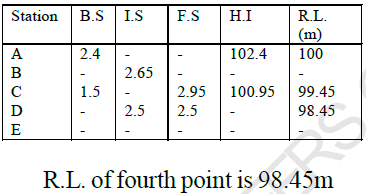Courses

# Surveying - 3

## 25 Questions MCQ Test GATE Civil Engineering (CE) 2022 Mock Test Series | Surveying - 3

Description
This mock test of Surveying - 3 for GATE helps you for every GATE entrance exam. This contains 25 Multiple Choice Questions for GATE Surveying - 3 (mcq) to study with solutions a complete question bank. The solved questions answers in this Surveying - 3 quiz give you a good mix of easy questions and tough questions. GATE students definitely take this Surveying - 3 exercise for a better result in the exam. You can find other Surveying - 3 extra questions, long questions & short questions for GATE on EduRev as well by searching above.
QUESTION: 1

### If the hypotenuse allowance for a ground A for 20m chain and for a ground B for 30m chain is same. The ratio of the gradient of ground A to that of ground B is

Solution: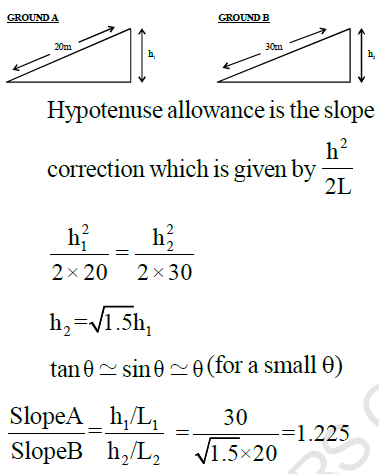*Answer can only contain numeric values
QUESTION: 2

### The bearings of two inaccessible stations S1 and S2 from a station S were obtained as 2400 and 1600 respectively. The independent coordinates of S1 and S2 are: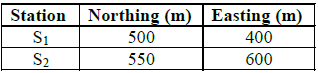The independent northing (in m) of station S3 is____ (Important - Enter the numerical value only in the answer)

Solution: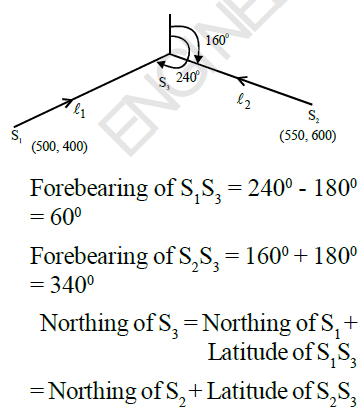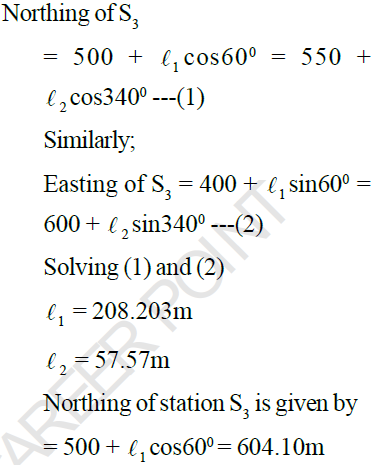*Answer can only contain numeric values
QUESTION: 3

### Linked Question: 3 & 4 The following reading refer to the reciprocal level taken between the two stations P and Q: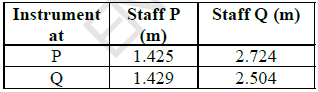Q. The true difference in elevation is _____ m. (Important - Enter the numerical value only in the answer)

Solution: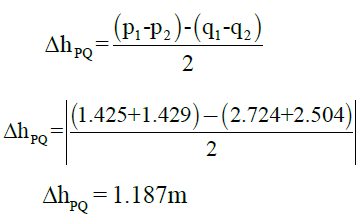*Answer can only contain numeric values
QUESTION: 4

Linked Question: 3 & 4

The following reading refer to the reciprocal level taken between the two stations P and Q:4. If the distance between the station is 1150m, find the collimation error (in m) per 150m distance._____m.

(Important - Enter the numerical value only in the answer)

Solution: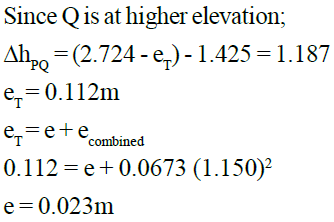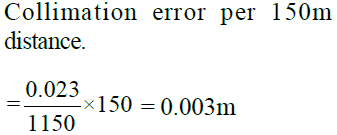*Answer can only contain numeric values
QUESTION: 5

The area of the plan of an old survey plotted to a scale of 1cm to 5m now measure 90cm2 as found by planimeter. The plan is found to have shrunk such that original line of 15cm length now measure only 14.5cm. A note on the plan also states that the 30cm chain used was 10cm too long. The true field area
of the survey will be____m2

(Important - Enter the numerical value only in the answer)

Solution: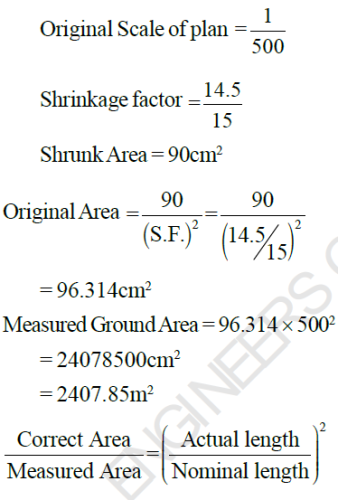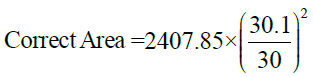Correct Area = 2423.93m2

QUESTION: 6

While operating a theodolite, the operation of bringing the vertical circle to the right of the observer, if originally it is to the left and vice versa is called as:

Solution:
QUESTION: 7

The whole circle bearing of side AB of an equillateral triangle ABC is 38045', then the bearing of the third
side CA of the triangle is:

Solution: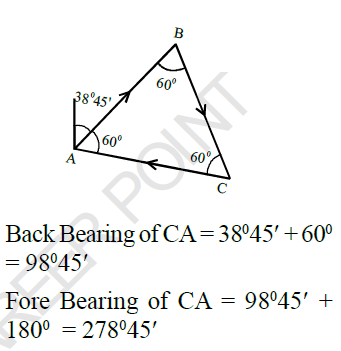QUESTION: 8

A line was measured with a steel tape which was exactly 30m at 200C at a pull of 100N. The measured length was 1500m. If the temperature during measurement was 400C and the pull applied was 150N, determine the correct length of line. Coefficient of thermal expansion of tape is 3.5 x 10-6 per0C, cross-sectional area of tape is 2.5mm2, Modulus of elasticity = 2.1 x105 N/mm2.

Solution: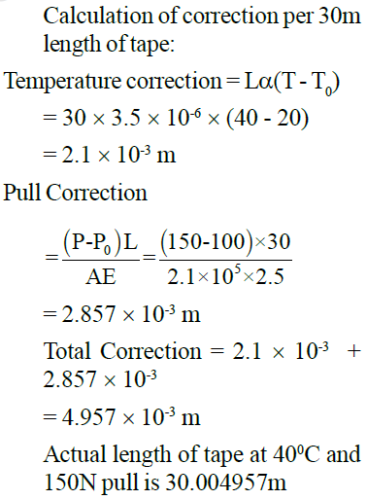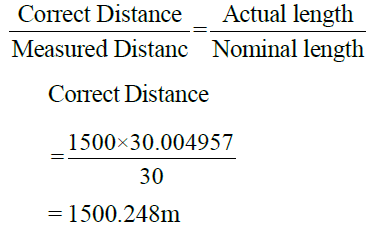QUESTION: 9

If the standard error in length of square (1m x 1m) is +0.1cm, the standard error in area will be

Solution: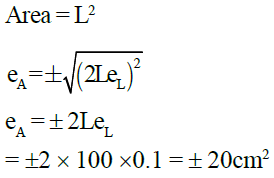QUESTION: 10

The most probable value of angle A from the following equations will be ___ degrees.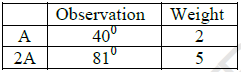Solution: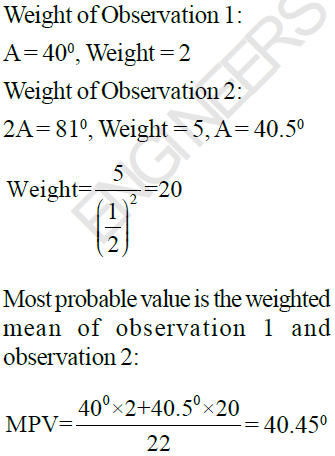*Answer can only contain numeric values
QUESTION: 11

The number of photographs of size (25cm x 25cm) required to cover the area of (20km x 16km) if the longitudinal overlap is 60% and side overlap is 30% will be: ____
Scale of the photograph is 1/15000

(Important - Enter only the numerical value in the answer)

Solution: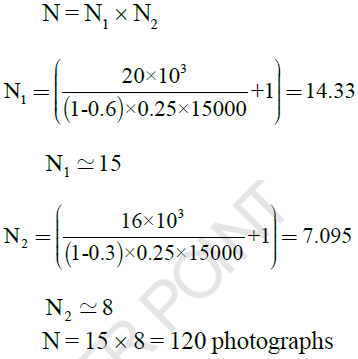*Answer can only contain numeric values
QUESTION: 12

The fore bearing and back bearing of line PQ were observed to be 205030' and 2400' respectively. It was known that station Q was free from local attraction. If the bearing of sun observed from station P at local noon is 3580. The true bearing of line PQ will be ____ .

(Important - Enter only the numerical value in the answer)

Solution: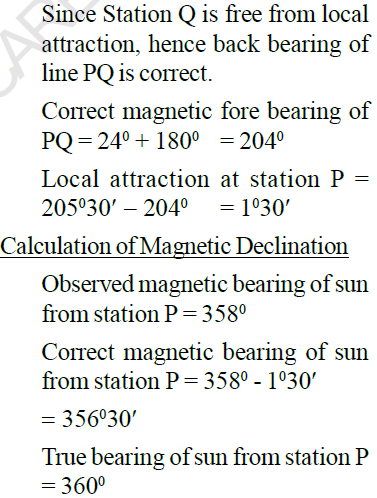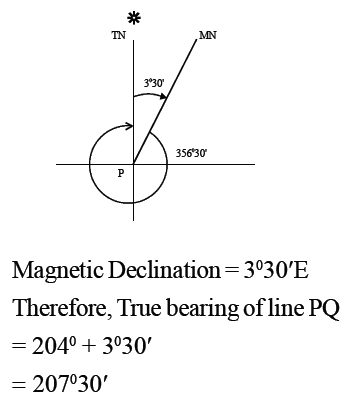QUESTION: 13

If the staff intercept on a staff located at 100m from the level for five divisions deviation of the bubble is 0.050m and if the length of one division of the bubble is 2mm, then the radius of curvature of the bubble tube is

Solution: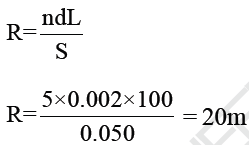*Answer can only contain numeric values
QUESTION: 14

A theodolite was setup in between  two towers X and Y. The distance between the two tower is 180m. The distance of theodolite station from tower X is 60m. Observations were taken from the theodolite to the top of tower X and tower Y and were recorded as 350 and 300 respectively, telescope focussed upward for both the cases. The R.L. of the top of the tower X is 180m. Calculate the R.L. of the top of the tower Y.____m

(Important - Enter only the numerical value in the answer)

Solution: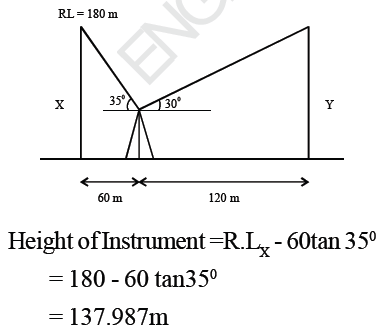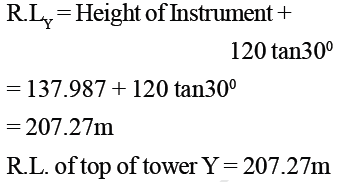QUESTION: 15

The coordinates of two points A and B are as follows: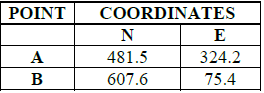Calculate the bearing of line AB.

Solution: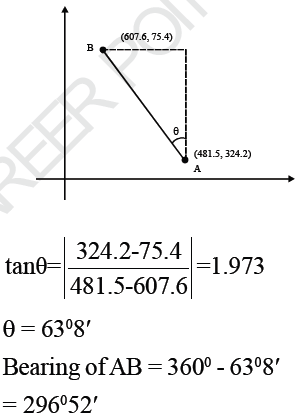QUESTION: 16

ABCD is a regular parallelogram plot of land, whose angle BAD is 600. If the bearing of the line AB is 400, then the bearing of the line CD is:

Solution: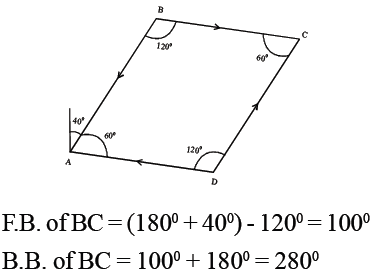F.B. of CD = 2800 - 600 = 2200

QUESTION: 17

A vertical photograph of chimney was taken from a elevation of 500m above the M.S.L. The elevation of the base of the chimney was 100m. If the relief displacement of the chimney was 52m and the radial distance of the image of the bottom of the chimney was 50m, the height of the chimney is:

Solution: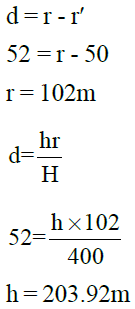*Answer can only contain numeric values
QUESTION: 18

In testing a dumpy level, following observations were recorded at staff station A and B: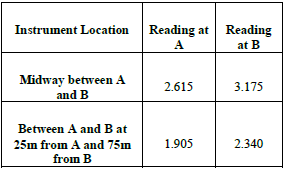Find the tan of angle of inclination of the line of collimation?

(Important - Enter the numerical value only in the answer)

Solution: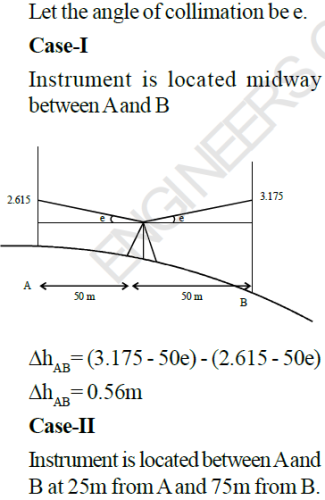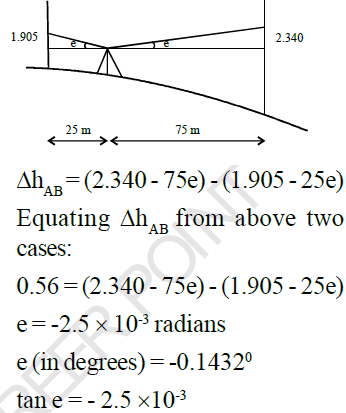QUESTION: 19

How long does it take for a GPS satellite to orbit around the Earth?

Solution:
QUESTION: 20

A survey line 2000m in length is measured with a 20m chain. If the accidental error in measurement is +0.04m for one chain length, calculate the total error involved when survey line is measured?

Solution: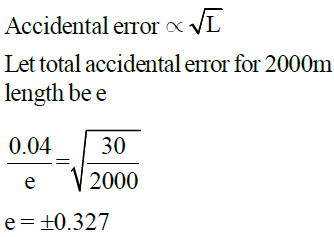QUESTION: 21

In a cross staff survey, the perpendicular offsets are taken on right and left of chain line PQ as shown in the figure - all values are in ‘meters’. The area enclosed by computed by trapezoidal method is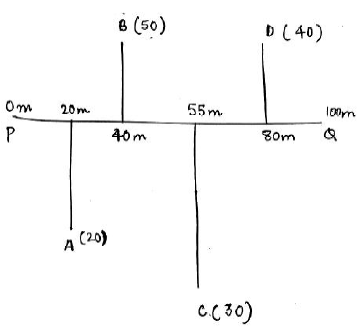Solution: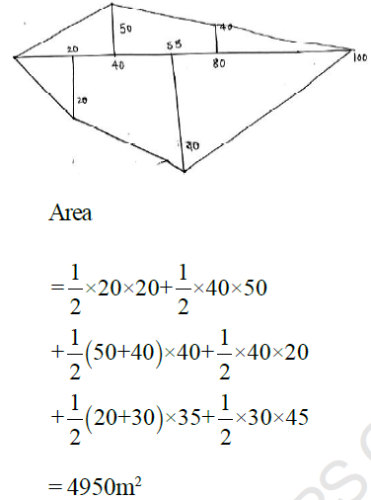*Answer can only contain numeric values
QUESTION: 22

Consider the following map having contours. The scale of map is 1:20,000 as written on the map. The map has shrunk such that 10cm line originally is now 9cm long. The alignment of a road at ruling gradient of 5% is to be fixed from the point P and beyond. What should be the radius of arc (in cm) with P as the centre to get the point of alignment of the next contour on the map? _________ cm

(Important - Enter the numerical value only in the answer)

Solution: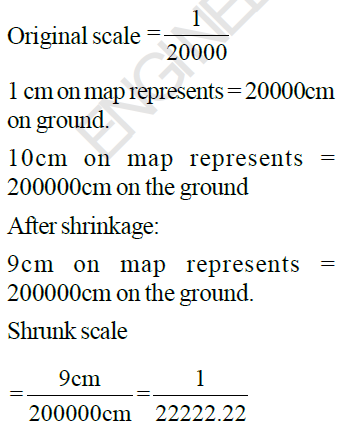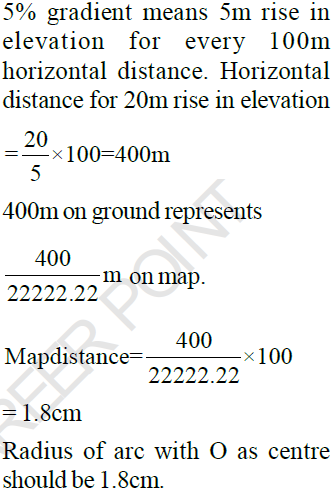QUESTION: 23

While carrying out adjustments of dumpy level, the following observations were made:

(i) Instrument exactly at midpoint of line AB.
Staff Reading at station A = 2.8 m
Staff Reading at sation B = 2.6 m

(ii) Instrument very near to station B.
Staff Reading at station A = 1.65 m
Staff Reading at station B = 1.85m

Find out the correct staff reading at A when the instrument is near the station B.

Solution: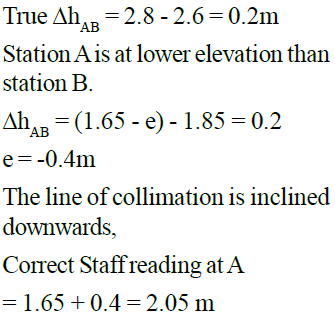*Answer can only contain numeric values
QUESTION: 24

A sailor standing on the deck of ship, just sees the bottom of light house. If the height of the sailor’s eye and the light beam at light house, above the sea level are 9m and 25m respectively, what is the distance between the sailor and the lighthouse ? ____ km

(Important - Enter the numerical value only in the answer)

Solution:

When the sailor sees the bottom of light house.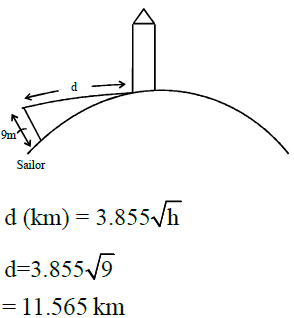QUESTION: 25

The following readings were taken with a dumpy level and a 4m levelling staff on a continuously sloping ground:

2.4, 2.65, 2.95, 1.5, 2.5, 2.75. The R.L. of first point is 100m.

The R.L. of fourth point is:

Solution: